Updating search results...

# 45 Results

View
Selected filters:
• CSDE - PublicRestricted Use
Rating
0.0 stars

Equitable and Inclusive Curriculum&nbsp;&nbsp;The CSDE believes in providing a set of conditions&nbsp;where learners are repositioned at the center of curricula planning and design.&nbsp;Curricula, from a culturally responsive perspective, require intentional planning for diversity, equity, and inclusion in the development of units and implementation of lessons. It is critical to develop a learning environment that is relevant to and reflective of students&rsquo; social, cultural, and linguistic experiences to effectively connect their culturally and community-based knowledge to the class. Begin by connecting what is known about students&rsquo; cognitive and interdisciplinary diversity to the learning of the unit. Opposed to starting instructional planning with gaps in students&rsquo; knowledge, plan from an asset-based perspective by starting from students&rsquo; strengths. In doing so, curricula&rsquo;s implementation will be grounded in instruction that engages, motivates, and supports the intellectual capacity of all students. Course Description:&nbsp;&nbsp;In Grade 6, instructional time should focus on four critical areas: (1) connecting ratio and rate to whole number multiplication and division and using concepts of ratio and rate to solve problems; (2) completing understanding of division of fractions and extending the notion of number to the system of rational numbers, which includes negative numbers; (3) writing, interpreting, and using expressions and equations; and (4) developing understanding of statistical thinking.&nbsp; Upon completion of this course students will have the ability to:Understand ratio concepts and use ratio reasoning to solve problems.&nbsp;Apply and extend previous understandings of multiplication and division to divide fractions by fractions.&nbsp;Multiply and divide multi-digit numbers and find common factors and multiples.&nbsp;Apply and extend previous understandings of numbers to the system of rational numbers.&nbsp;Apply and extend previous understandings of arithmetic to algebraic expressions.&nbsp;Reason about and solve one-variable equations and inequalities.&nbsp;Represent and analyze quantitative relationships between dependent and independent variables.&nbsp;Solve real-world and mathematical problems involving area, surface area, and volume.&nbsp;Develop understanding of statistical variability.&nbsp;Summarize and describe distributions.Aligned Core Resources:&nbsp;&nbsp;Core resources is a local control decision.&nbsp; Ensuring alignment of resources to the standards is critical for success.&nbsp;&nbsp;There are tools that are available to assist in evaluating alignment, such as CCSSO&rsquo;s Mathematics Curriculum Analysis Project and Student Achievement Partner&rsquo;s Instructional Materials Evaluation Tool.&nbsp; In addition&nbsp;EdReports&nbsp;and Louisiana Believes are two sources of completed reviews for a variety of resources.&nbsp; Connecticut is currently working on providing additional alignment guidance for the most frequently used resources across the state.&nbsp;Additional Course Information:&nbsp;&nbsp;Major work of&nbsp;Grade 6 mathematics&nbsp;focuses on ratios and proportional relationships and early expressions and equations.&nbsp; Fluencies required upon completion of grade 6 include multi-digit division and multi-digit decimal operations.&nbsp;Habits of Mind/SEIH/Transferable Skills Addressed in the Course:&nbsp;The&nbsp;Standards for Mathematical Practice describe the thinking processes, habits of mind, and dispositions that students need to develop a deep, flexible, and enduring understanding of mathematics. They describe student behaviors, ensure an understanding of math, and focus on developing reasoning and building mathematical communication. Therefore,&nbsp;the following should be addressed throughout the course:&nbsp;Make sense of problems &amp; persevere in solving them&nbsp;Reason abstractly &amp; quantitatively&nbsp;Construct viable arguments &amp; critique the reasoning of others&nbsp;Model with mathematics&nbsp;Use appropriate tools strategically&nbsp;Attend to precision&nbsp;Look for &amp; make use of structure&nbsp;Look for &amp; express regularity in repeated reasoning&nbsp;&nbsp;

Subject:
Mathematics
Material Type:
Full Course
Provider:
CT State Department of Education
Provider Set:
CSDE - Public
09/21/2021Restricted Use
Rating
0.0 stars

Unit Overview/Summary - FOCUS:&nbsp;&nbsp;This unit focuses on the&nbsp;Equations and Expressions&nbsp;domain.&nbsp; Learning in this unit will enable students to:&nbsp;&bull;&nbsp;Reason about and solve one-variable equations and inequalities.&nbsp;&bull; Represent and analyze quantitative relationships between dependent and independent variables.&nbsp;All of the learning in this unit is major work of the grade.&nbsp;

Subject:
Mathematics
Provider:
CT State Department of Education
Provider Set:
CSDE - Public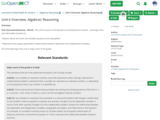Restricted Use
Rating
0.0 stars

Unit Overview/Summary - FOCUS:&nbsp;&nbsp;This unit focuses on the&nbsp;Equations and Expressions&nbsp;domain.&nbsp; Learning in this unit will enable students to:&nbsp;&bull;&nbsp;Reason about and solve one-variable equations and inequalities.&nbsp;&bull; Represent and analyze quantitative relationships between dependent and independent variables.&nbsp;All of the learning in this unit is major work of the grade.&nbsp;

Subject:
Mathematics
Material Type:
Unit of Study
Author:
Connecticut Department of Education
10/12/2021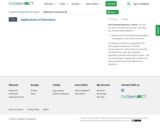Restricted Use
Rating
0.0 stars

Unit Overview/Summary - FOCUS:&nbsp;&nbsp;This unit focuses on the Geometry domain.&nbsp; Learning in this unit will enable students to:&nbsp;Solve real-world and mathematical problems involving area, surface area and volume.&nbsp;The Geometry domain is a supporting cluster within grade 6 mathematics.&nbsp; Therefore the&nbsp;learning of the content of this unit should be connected to the major work of grade 6, specifically, arithmetic with&nbsp;rational numbers.&nbsp; This unit also provides an opportunity to address the fluency requirement of multi-digit decimal operations.&nbsp;

Subject:
Mathematics
Provider:
CT State Department of Education
Provider Set:
CSDE - Public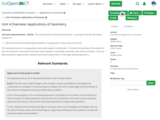Restricted Use
Rating
0.0 stars

Unit Overview/Summary - FOCUS:&nbsp;&nbsp;This unit focuses on the Geometry domain.&nbsp; Learning in this unit will enable students to:&nbsp;Solve real-world and mathematical problems involving area, surface area and volume.&nbsp;The Geometry domain is a supporting cluster within grade 6 mathematics.&nbsp; Therefore the&nbsp;learning of the content of this unit should be connected to the major work of grade 6, specifically, arithmetic with&nbsp;rational numbers.&nbsp; This unit also provides an opportunity to address the fluency requirement of multi-digit decimal operations.&nbsp;

Subject:
Mathematics
Material Type:
Unit of Study
Author:
Connecticut Department of Education
10/12/2021Restricted Use
Rating
0.0 stars

Unit Overview/Summary - FOCUS:&nbsp;This unit focuses on the Number Systems domain&nbsp;and provides an opportunity&nbsp;to apply number systems content within the context of geometry.&nbsp;&nbsp;Learning in this unit will enable students to:&nbsp;&bull;&nbsp;&nbsp;&nbsp; Apply and extend previous understandings of multiplication and division to divide fractions by fractions.&nbsp;&bull;&nbsp;&nbsp;&nbsp; Compute fluently with multi-digit numbers and find common factors and multiples&nbsp;&bull;&nbsp;&nbsp;&nbsp; Solve real-world and mathematical problems involving area, surface area, and volume.&nbsp;All of the learning in this unit is major work of the grade.&nbsp;

Subject:
Mathematics
Provider:
CT State Department of Education
Provider Set:
CSDE - Public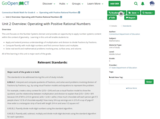Restricted Use
Rating
0.0 stars

This unit focuses on the Number Systems domain&nbsp;and provides an opportunity&nbsp;to apply number systems content within the context of geometry.&nbsp;&nbsp;Learning in this unit will enable students to:&nbsp;Apply and extend previous understandings of multiplication and division to divide fractions by fractions.Compute fluently with multi-digit numbers and find common factors and multiples&nbsp;Solve real-world and mathematical problems involving area, surface area, and volume.&nbsp;All of the learning in this unit is major work of the grade.&nbsp;

Subject:
Mathematics
Material Type:
Unit of Study
Author:
Connecticut Department of Education
09/13/2021Restricted Use
Rating
0.0 stars

Unit Overview/Summary - FOCUS:&nbsp;This unit focuses on the Ratios and Proportional Relationships domain.&nbsp; Learning in this unit will enable students to:&nbsp;&bull;&nbsp;&nbsp;&nbsp; Understand ratio concepts and use ratio reasoning to solve problems.&nbsp;All of the learning in this unit is major work of the grade.&nbsp;

Subject:
Mathematics
Provider:
CT State Department of Education
Provider Set:
CSDE - Public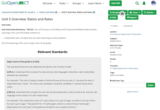Restricted Use
Rating
0.0 stars

Unit Overview/Summary - FOCUS:&nbsp;This unit focuses on the Ratios and Proportional Relationships domain.&nbsp; Learning in this unit will enable students to:&nbsp;&bull;&nbsp;&nbsp;&nbsp; Understand ratio concepts and use ratio reasoning to solve problems.&nbsp;All of the learning in this unit is major work of the grade.&nbsp;

Subject:
Mathematics
Material Type:
Unit of Study
Author:
Connecticut Department of Education
10/12/2021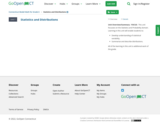Restricted Use
Rating
0.0 stars

Unit Overview/Summary - FOCUS:&nbsp;&nbsp;This unit focuses on the&nbsp;Statistics and Probability&nbsp;domain.&nbsp; Learning in this unit will enable students to:&nbsp;Develop understanding of statistical variability.&nbsp;Summarize and describe distributions.&nbsp;All of the learning in this unit is additional work of the grade.&nbsp;

Subject:
Mathematics
Provider:
CT State Department of Education
Provider Set:
CSDE - Public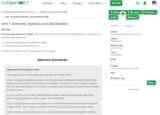Restricted Use
Rating
0.0 stars

Unit Overview/Summary - FOCUS:&nbsp;&nbsp;This unit focuses on the&nbsp;Statistics and Probability&nbsp;domain.&nbsp; Learning in this unit will enable students to:&nbsp;Develop understanding of statistical variability.&nbsp;Summarize and describe distributions.&nbsp;All of the learning in this unit is additional work of the grade.&nbsp;

Subject:
Mathematics
Material Type:
Unit of Study
Author:
Connecticut Department of Education
10/12/2021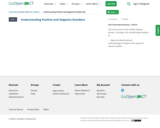Restricted Use
Rating
0.0 stars

Unit Overview/Summary - FOCUS:&nbsp;&nbsp;This unit focuses on the Number Systems domain.&nbsp; Learning in this unit will enable students to:&nbsp;&bull;&nbsp;&nbsp;&nbsp; Apply and extend previous understanding&nbsp;of&nbsp;numbers to the system of rational numbers&nbsp;

Subject:
Mathematics
Provider:
CT State Department of Education
Provider Set:
CSDE - Public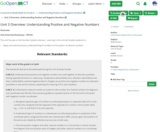Restricted Use
Rating
0.0 stars

Unit Overview/Summary - FOCUS:&nbsp;&nbsp;This unit focuses on the Number Systems domain.&nbsp; Learning in this unit will enable students to:&nbsp;&bull;&nbsp;&nbsp;&nbsp; Apply and extend previous understanding&nbsp;of&nbsp;numbers to the system of rational numbers&nbsp;

Subject:
Mathematics
Material Type:
Unit of Study
Author:
Connecticut Department of Education
10/12/2021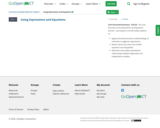Restricted Use
Rating
0.0 stars

Unit Overview/Summary - FOCUS:&nbsp;&nbsp;This unit focuses on the Expressions and Equations domain.&nbsp; Learning this unit will enable students to:&nbsp;Apply and extend previous understandings of arithmetic to algebraic expressions.&nbsp;Reason about and solve one-variable equations and inequalities.&nbsp;Represent and analyze quantitative relationships between dependent and independent variables.&nbsp;&nbsp;

Subject:
Mathematics
Provider:
CT State Department of Education
Provider Set:
CSDE - Public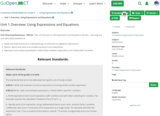Restricted Use
Rating
0.0 stars

Unit Overview/Summary - FOCUS:&nbsp;&nbsp;This unit focuses on the Expressions and Equations domain.&nbsp; Learning this unit will enable students to:&nbsp;Apply and extend previous understandings of arithmetic to algebraic expressions.&nbsp;Reason about and solve one-variable equations and inequalities.&nbsp;Represent and analyze quantitative relationships between dependent and independent variables.&nbsp;&nbsp;

Subject:
Mathematics
Material Type:
Unit of Study
Author:
Connecticut Department of Education
10/12/2021Restricted Use
Rating
0.0 stars

Subject:
Mathematics
Material Type:
Full Course
Provider:
CT State Department of Education
Provider Set:
CSDE - Public
10/12/2021Restricted Use
Rating
0.0 stars

Unit Overview/Summary - FOCUS:&nbsp;This unit focuses on Expressions and Equations. Learning this unit will enable students to:&nbsp;Solve real-life and mathematical problems using numerical and algebraic expressions and equations.&nbsp;Use properties of operations to generate equivalent expressions.&nbsp;

Subject:
Mathematics
Provider:
CT State Department of Education
Provider Set:
CSDE - Public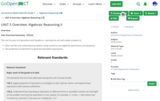Restricted Use
Rating
0.0 stars

Unit Overview/Summary - FOCUS:&nbsp;This unit focuses on Expressions and Equations. Learning this unit will enable students to:&nbsp;Solve real-life and mathematical problems using numerical and algebraic expressions and equations.&nbsp;Use properties of operations to generate equivalent expressions.&nbsp;

Subject:
Mathematics
Material Type:
Unit of Study
Author:
Connecticut Department of Education
10/25/2021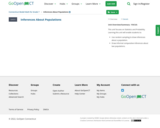Restricted Use
Rating
0.0 stars

Unit Overview/Summary - FOCUS:&nbsp;This unit focuses on Statistics and Probability. Learning this unit will enable students to:&nbsp;Use random sampling to draw inferences about a population.&nbsp;Draw informal comparative inferences about two populations.&nbsp;

Subject:
Mathematics
Provider:
CT State Department of Education
Provider Set:
CSDE - Public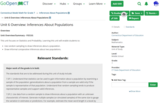Restricted Use